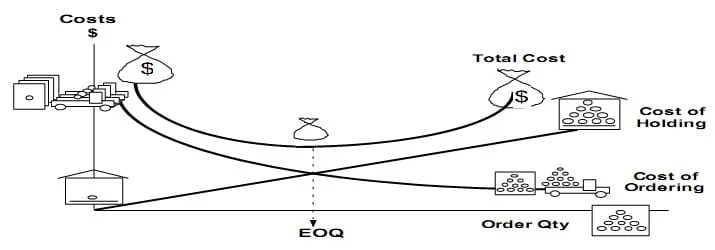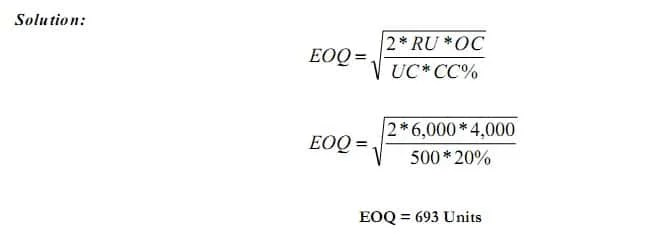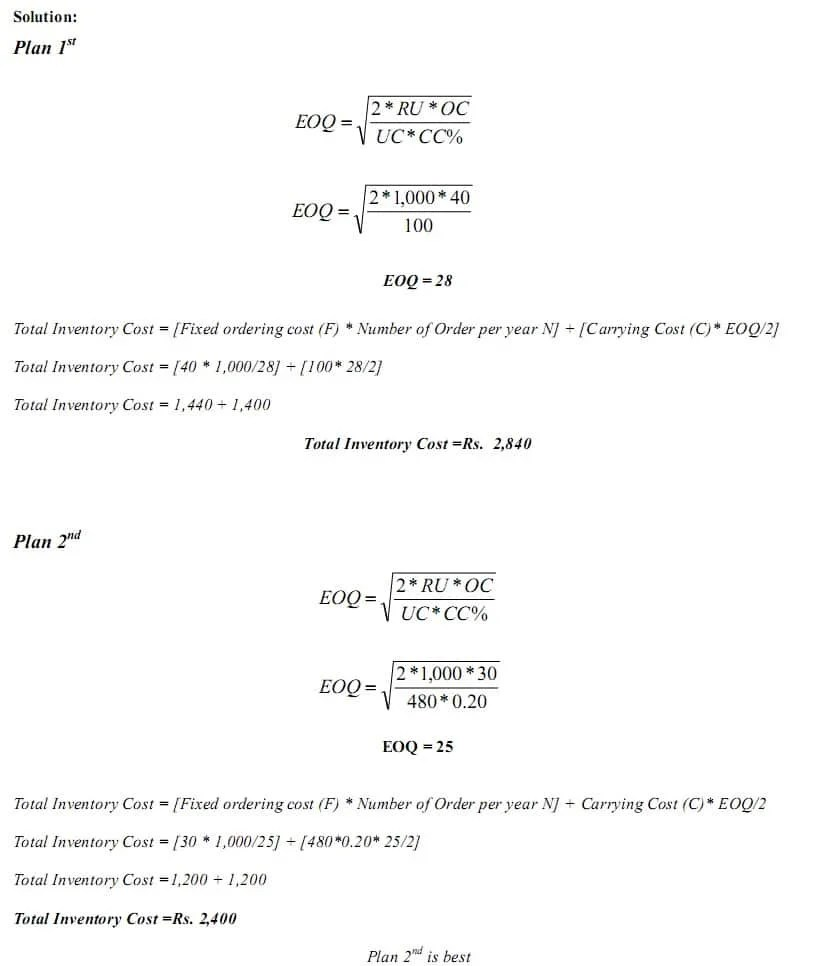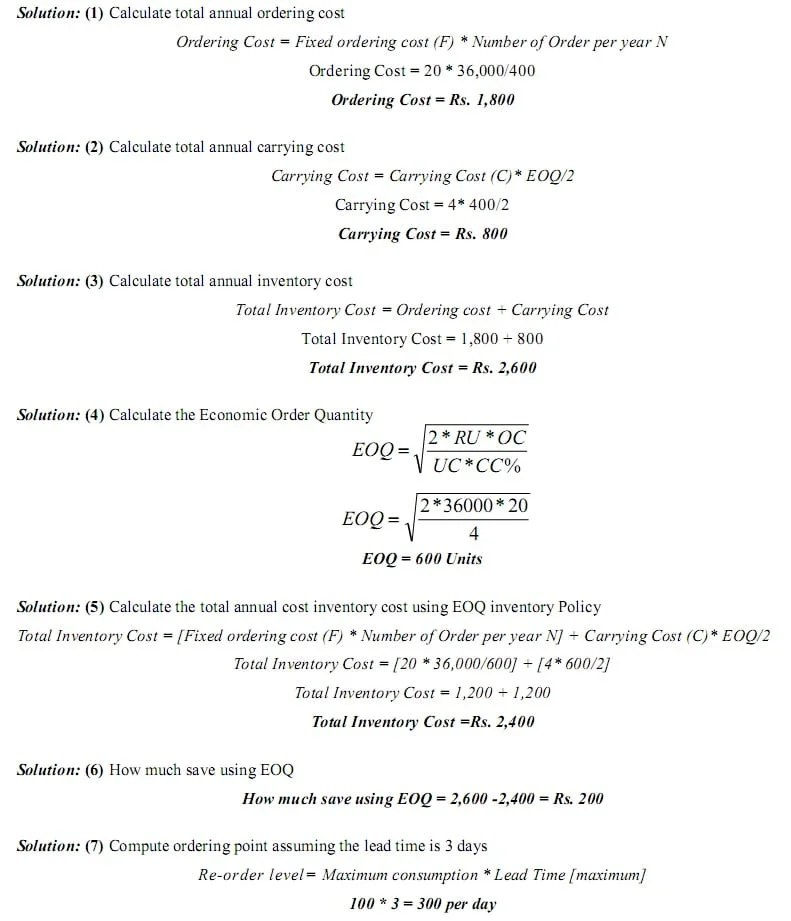Select Page

# Economic Order Quantity

Previous Lesson: Inventory Management

Next Lesson:

Economic Order Quantity is the level of inventory that minimizes the total inventory holding costs and ordering costs. It is one of the oldest classical production scheduling models. Economic order quantity refers to that number (quantity) ordered in a single purchase so that the accumulated costs of ordering and carrying costs are at the minimum level. In other words, the quantity that is ordered at one time should be so, which will minimize the total of. Cost of placing orders and receiving the goods, and Cost of storing the goods as well as interest on the capital invested.The economic order quantity can be determined by the following simple formula:

#### FormulaEOQ = Economic Order Quantity,

RU = Annual Required Units,

OC    = Ordering Cost for one Unit

UC = Inventory Unit Cost,

CC = Carrying Cost as %age of Unit Cost

>>> Practice

## Example 1:

Demand for the Child Cycle at Best Buy is 500 units per month. Best Buy incurs a fixed order placement, transportation, and receiving cost of Rs. 4,000 each time an order is placed. Each cycle costs Rs. 500 and the retailer has a holding cost of 20 percent. Evaluate the number of computers that the store manager should order in each replenishment lot?>> Practice

## Example 2:

ABC Ltd. uses EOQ logic to determine the order quantity for its various components and is planning its orders. The Annual consumption is 80,000 units, Cost to place one order is Rs. 1,200, Cost per unit is Rs. 50 and carrying cost is 6% of Unit cost. Find EOQ, No. of order per year, Ordering Cost and Carrying Cost and Total Cost of Inventory.>> Question and Answers:  Economic Order Quantity Problems and Solutions

## Example 3:

Midwest Precision Control Corporation is trying to decide between two alternate Order Plans for its inventory of a certain item. Irrespective of the plan to be followed, demand for the item is expected to be 1,000 units annually. Under Plan 1st, Midwest would use a teletype for ordering; order costs would be Rs. 40 per order. Inventory holding costs (carrying cost) would be Rs. 100 per unit per annum. Under Plan 2nd order costs would be Rs. 30 per order. And holding costs would 20% and unit Cost is Rs. 480. Find out EOQ and Total Inventory Cost than decide which Plan would result in the lowest total inventory cost?>> Practice Economic Order Quantity Problems and Solutions

## Example 4:

A local TV repairs shop uses 36,000 units of a part each year (A maximum consumption of 100 units per working day). It costs Rs. 20 to place and receive an order. The shop orders in lots of 400 units. It cost Rs. 4 to carry one unit per year of inventory.

Requirements:

(1) Calculate total annual ordering cost

(2) Calculate total annual carrying cost

(3) Calculate total annual inventory cost

(4) Calculate the Economic Order Quantity

(5) Calculate the total annual cost inventory cost using EOQ inventory Policy

(6) How much save using EOQ

(7) Compute ordering point assuming the lead time is 3 days>> Practice Economic Order Quantity Problems and Solutions

### Related Topics

Inventory Management

Inventory Management MCQs

Inventory Management Problems

Economic Order Quantity MCQ

Economic Order Quantity Problems

### More Interest

Labor Cost

Payroll Systems

Labor Cost MCQs

Labor Cost Problems

Inventory Valuation

## References

Mukharji, A., & Hanif, M. (2003). Financial Accounting (Vol. 1). New Delhi: Tata McGraw-Hill Publishing Co.

Narayanswami, R. (2008). Financial Accounting: A Managerial Perspective. (3rd, Ed.) New Delhi: Prentice Hall of India.

Ramchandran, N., & Kakani, R. K. (2007). Financial Accounting for Management. (2nd, Ed.) New Delhi: Tata McGraw Hill.

1. Ethio Company predicts that it will use 50,000 units of materials during the year. The expected daily usage is 250 units there is an expected lead time of six days and a safety stock of 700 units. The material is expected to cost Birr 8 per unit. Ethio Company anticipates that it will cost Birr 70 to place each order. The annual caring cost is Birr 0.30 per unit. So, how to compute the order point? Determine the most economical order quantity by use of the formula? And calculate the cost of order and carrying at the EOQ point.

2. A building material shop owner in Roorkee is going through the last year’s inventory cost of cement sacks, a sack of cement costs 500 Rs. The average monthly demand last year was 1250 units, the cost per order, including the transport was 1200 Rs and annual unit holding cost was 20% of material cost. Last year he ordered the lots of 500 sacks per order.
What was the total ordering cost for the last year?
what was the total inventory cost last year?
how much lot size should the shop owner order to minimize total inventory cost assuming a constant demand pattern?
how much the shop owner will save in total inventory cost (in Rs.) in the coming year?

3. Chico’s Chili (CC) is well known for making the best chili in Chicago. Chico makes a variety of different types of chili and customers can combine it together with any sides they like. Chico’s chili is so popular that it uses about 120 kgs of ground beef per day and he is open 348 days (50 weeks) per year.

Chico has been ordering beef once per week and has determined that it takes a ½ hour to place an order. His hourly rate is \$40. Chico has also determined that inventory spoilage is 1% and his interest rate on debt is 8%. Chico pays \$10.00 per kg for his ground beef. The lead time to place a beef order up to delivery is 3 days.

Chico has just learned about something called the EOQ and now thinks if he might be able to save money by changing his order pattern. Please calculate the following:
1. Calculate how many kgs Chico purchases in a year .

2. Calculate Chico’s current Order Quantity .

3. What is the cost of placing one order?

4. What is the Carrying Cost of one kg of beef?

5. Calculate the current Total Annual Inventory Cost.

6. Calculate Chico’s economic order quantity (EOQ).

7. Using the EOQ, recalculate the Total Inventory Annual Costs.
/

4. Ethio Company predicts that it will use 50,000 units of materials during the year. The expected daily usage is 250 units there is an expected lead time of six days and a safety stock of 700 units. The material is expected to cost Birr 8 per unit. Ethio Company anticipates that it will cost Birr 70 to place each order. The annual caring cost is Birr 0.30 per unit. So, how to compute the order point? Determine the most economical order quantity by use of the formula? And calculate the cost of order and carrying at the EOQ point.

• Compute order point

• give answer for this quation

6. Horizon campany makes and sells specially gear for the retail automobile after market. The has estimated the annual demand for a component (gear) is 1000 units next year, with an average daily demand production most efficient at 8 units per a day. In connection with production , the Experience of the company shows that the set up cost is 10 per order and the holding cost is birr 0.50 per gear per year. (Note; there are 250 working days in a year).
1,what is Economic order quantity?
2,How many production runs will be made per year?
3,What will be the maximum inventory levele?
4,The time gap between two production run is
5,How many days take a single production run to be finished?
6,For how many days in the year the production unit will be idle?
7,Total minimum Stocking cost of the Company is??
8, At break event point???

You are a manufacturer of tennis balls in the Mumbai Suburbs. Recently, you got an
order to supply 1200 units of the same on a monthly basis. The cost of carrying an
inventory of such tennis balls is 1.80 per unit on yearly basis. The production process
requires a setup cost on a per run basis of Rs 1000.

Compute:
a. The EOQ, and define the need of computing the EOQ
b. The Optimum number of orders and optimum period of supply

8. From the following information provided by Alfa Manufacturing Ltd, prepare a
statement of equivalent units.

Particulars Quantity

Opening stock of inventory
(60 percent complete) – 500

Units introduced during the year – 10000

Closing inventory of stock
(completion percentage, 40% complete)- 200

9. 2. Following information relating to a type of raw material is available: Annual demand 2,400 units
Unit price​RO 2,400
Ordering cost per order RO .400
Storage cost: 2 % per annum
Interest rate: 10% per annum
Calculate EOQ and the number of orders that are required to be placed during the year.

• EOQ=√((2×2400×400)/(2%×10%)
= 30984

10. A car manufacturing company produces 450 cars in one month. It buys the engines for cars from a supplier at a cost of ₦20 per engine. The company’s inventory carrying cost is estimated to be 15% of cost and the ordering is ₦50 per order. You are required to:

i. Compute the average annual ordering cost.

ii. Compute the average inventory

Please assist me with a solution to this question

• i) EOQ= √(2×5400×50)÷3

EOQ=424 units

ii) Average Inventory=EOQ/2

=424/2= 212 units

• Ordering cost= no.orders×max quantity
=20×450(12)
= 108,000

11. I. Calculate the Economic Order Quantity and number of orders to be placed in a year for tablet clarithromycin 500 mg used in a tertiary car,e center.

Annual consumption of tablet clarithromycin 500 mg is 470 no. and it is usually purchased at the cost of Rs. 150 (tab 4s). The buying cost per order is Rs. 170. Its carrying and storage cost is Rs. 112.

12. The Florida company has obtained the following costs and other data pertaining to one of its materials:
Workign days per year 250
Normal use per day 500 units
Max. use per day 600 units
Min. use per day 100 units
variable cost of placing one order \$ 36
variable carrying cost per unit per year \$ 1

Required:

a) Economic Order Quantity

b) Safety Stock

c) Order Point

13. sorry may you help me that question;

1. An item has a monthly demand of 2500 units and each item costs \$ 10.00 to purchase it. the cost of placing order per unit is \$ 10.00 while holding cost are estimated to be 2% of the stock value. if the organization works for 10 months in a year. calculate the followings
(a) Economic Order Quantity
(b) number of orders costs
(c) total ordering cost
(d) average inventory level
(c) the re-order point if lead time 5 days

• Why economic order quantity does not have quantity discount?

14. Can anybody help with solutions to the following questions?

Problem 1:
A large firm uses an average of 40 boxes of copier paper a day. The firm operates 260 days per year. Storage and handling costs for the paper are \$30 a year per box, and it costs approximately \$60 to order and receive a shipment of paper.
a) What order size would minimize the sum of annual ordering and carrying cost?
b) Compute the total annual cost using your order size from part a.
c) The office manager is currently using an order size of 200 boxes. The partners of the firm expect the office to be managed ‘in a cost-efficient manner’. Would you recommend that the office manager use the optimal order size instead of 200 boxes?
Problem 2:
A jewelry firm buys semiprecious stones to make bracelets and rings. The supplier quotes a price of \$8 per stone for quantities of 600 stones or more, \$9 per stone form orders of 400 to 599 stones, and \$10 per stone for lesser quantities. The jewelry firm operates 200 days per year. Usage rate is 25 stones per day and ordering costs are \$48.
a) If carrying costs are \$2 per year for each stone, find the order quantity that will minimize total annual cost.
b) If annual carrying costs are 30 percent of unit cost, what is the optimal order size?
c) If lead time is six working days, at what point should the company reorder?

• A. Eoq is 490
B. 421

15. An assistant manager is reviewing the costs associated with one of the store’s better-selling products. The data available follows.
Demand = 400 units/year

Order cost = K25/order

Holding cost = 50n/unit/year

a) They currently order a one-year supply. What are the total annual ordering cost and holding cost for this order size? (7 Marks)

b) What would be the EOQ and its associated ordering and holding costs?

16. As an inventory manager, you must decide on the order quantity for an item. Its annual demand is 1,000 units. Ordering costs are K50 each time an order is placed, and the holding cost is 25 percent of the per-unit price. Your supplier provided the following price schedule.

Quantity

Price per Unit

1–199

K10.00

200–499

K 9.80

500 or more

K 9.60

What ordering-quantity policy do you recommend?

17. Out Camping is a manufacturer of supplies and has several divisions, one of which is the tent division that produces the deluxe tent Away From Home. Fourteen thousand of these tents are made each year. This model of tent incorporates two zipping doors in each model. Zippers for these doors are purchased from Zippy Zippers. Out Camping began this year with 500 zippers in inventory, but is now adopting an economic order quantity approach to inventory. A review of last year’s records reveals the following information concerning each order placed with Zippy Zippers: variable labor— \$12; variable supplies—\$1; fixed computer costs—\$4; fixed office expenses—\$2; fixed mailing expenses— \$2; and fixed supplies—\$1. No differences are expected this year. Last year, the cost of carrying one zipper in inventory for the entire year was \$1, but Out Camping has recently negotiated a new lease on its warehouse, and the cost of carrying each zipper is now expected to increase by 25 cents. Out Camping also found that it took 7 days from the time an order was sent to Zippy until the zippers were received.

Required: Show your solutions in a detailed format.

a. Compute economic order quantity.
b. Compute reorder point. Presume Out Camping has a desire to reduce its ending inventory to one-half of this year’s beginning amount and that the company produces the Away From Home model 140 days each year.

18. Hello.
I need help to answer this question

A certain item cost \$235 per ton. The monthly requirements are 5 tons and each time the stock is \$1000. The cost of carrying inventory has been estimated at 10% of the value of the stock per year.

(a) what is the optimal total cost.

(b) what is optimal order quantity

(c) Determine the optimal time between two orders

(d) what is the optimal number of orders

19. Help me Solve it please

Dell computer stocks and sells laptops. Each time to places an order it costs the store \$645 with the manufacturer for laptops. In the current year, the company has ordered laptops for the three times. Per month, carrying cost of the laptops in inventory is \$13. Estimated annual demand for the laptops will be XYZ units. Determine the total minimum inventory cost, Number of orders per year and length of order cycle.

a manufacturing company uses 40000 kilograms of a raw material evenly over a period. the material is purchased at rm2.50 per kilogram. the cost of placing an order is rm120 and the cost of holding 1 kilogram of inventory for the period is 15% of the purchase cost.

a)calculate the economic order quantify (eoq)
b)calculate the total holding of the raw material in the period if the order quantity is 6,000 kilograms and the buffer inventory is 2,000 kilograms.

• Just consider that the raw materials in kg are units (it could make it easier for you to translate to the inventory formulas).

a) The EOW is sqrt[(2x40000x120)/(0.15×2.5)] ~ 5060

b) The total holding cost is the sum of the normal holding cost with the buffer (safety stock).

normal holding cost = (6000/2)x0.375 = 1125
Safety stock (buffer ) = 2000×0.375 = 750

Total holding cost = 1125 + 750 = 1875

21. Please I need an urgent help with the following problem:

The demand for an item is 44,000 units per annum, the ordering cost is \$50 per order. The holding cost per item is \$2.5 per annum and the price per unit of the item is \$10. The supplier offers a discount rate of 3% for orders between 10,000 to 31,999 and 8% discount rate for orders of 32,000 and above.

You’re required to determine the best quantity to order

22. ABC Corp. currently places orders for a particular stock item at quarterly intervals. Information concerning this items is as follows: P10 Cost of placing an order 25,000 units Annual demand P0.50 Carrying cost per unit What annual cost saving would result if ABC used the economic order quantity for order sizes instead of their current policy?

23. 2) The Star Equipment Company purchases 54,000 bearing assemblies each year at a unit cost of \$40.00. The holding cost is \$9.00 per unit per year, and the ordering cost is \$20.00.
(a) What is the economic order quantity?
(b) How many orders should be placed per year?
(c) If the lead time is one (01) month, what is the re-order point for the assemblies?

• (a) 490
(b) 110
(c) 4500

• A company requires 1000 units of raw material per month. Ordering cost is Rs 150 per order. Carrying cost in addition to Rs. 3 per unit is estimated to be 20% of average inventory per year. Cost of raw material is Rs. 10 per unit. Find EOQ, the number of orders in a year, and the total Inventory cost.

Need help with this prblm!

• If annual requirements is 2000 units, ordering cost is 10% per order and carrying cost is 0.16 per year per unit of average inventory value. Suppose the supplier allows to pay 1/= per unit. If you buy bellow 1000, but if you buy above 1000 unit but below 2000 you will get 5% purchase discount (0.95/=) and if you buy 2000 unit or above you will get 7% purchase discount (0.93/=) what unit should you purchase? I need the answer please.

• A firm has \$ 4 per year carrying cost on each unit of inventory and annual usage of 50,000 units. Ordering cost is \$ 100 per order. Calculate economic ordering quantity, total cost of inventory, annual ordering cost and annual carrying cost?

I need how to solve this question

website. Keep up the great writing.
King regards,
Balle Zacho

25. Thanks man. Very useful.

26. hardware shop in Gweru sells 2500 brackets in a year and the sales is relatively constant throughout the
year. These brackets are purchased from a supplier from Harare for \$15 each, and lead time is two days.
The holding cost per bracket per year is \$1.5(or 10% of the unit cost) and the ordering cost per order is
\$18. 75. There are 250 working days per year.
1. What is the EOQ?
2. Given the EOQ, what is the aver

• EOQ = 250 Brackets
TOTAL COST = \$ 375

27. I pass through the notice i understand

• Thank you

• I need help on a Question Sir